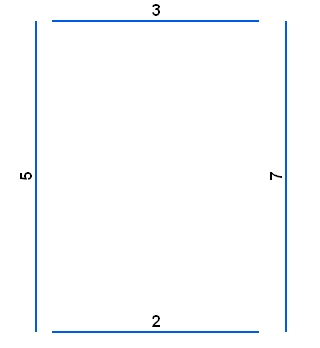Go to ET GeoWizards 12.x User Guide

Random Points On Polylines

Go to ToolBox Implementation

Generates random points located on the polylines of the input polyline dataset. The number of points per polyline can be constant or different for each polyline - based on the values in a numeric field of the input polyline feature class."

Inputs:

• A polyline feature class
• The number of points per polyline can be input in one of the following ways
• A numeric field which values will be used to get the number of points to be generated per polyline.
• A constant number
• Optional: The maximum number of points per polyline. This parameter is to enforce max number of points if a field is used for getting the number of points to be generated. For example if a field is selected and the values in this field range from 5 to 50,000. And the maximum number of points is set to 100, on the polylines that have values > 100 in the field only 100 points will be generated. The default value is 500.
• Optional: Minimum Distance from boundary - no point will be generated that is closer than this tolerance to the ends of the polyline (introduced in version 10.2).

Outputs:

• New Point feature class.
• The following fields are added to the attribute table of the resulting feature class.
• [ET_ID] - the ID of the original polyline
• [ET_X] - the X coordinate of the point
• [ET_Y] - the Y coordinate of the point
• [ET_Station] - the absolute position of the point along the polyline (in the units of the spatial reference of the input point feature class)

Note: Be careful with assigning the number of points per polyline. The function will try to create N unique points on the polyline and if the number of points allocated is too large, might be very slow or even fall into an indefinite loop.

Illustration:

 Original polylines labeled with the values in field to be used as a source for getting the number of points to be generated The resulting point datasetCommand line syntax

ET_GPRandomPointsOnPolylines<input_dataset>  <out_feature class> {number_points_field} {number_points}

Parameters

Expression Explanation
<input_dataset> A Plyline feature class or feature layer
<out_feature class> A String - the full name of the output feature class.
{number_points_field} Optional.  A String numeric field which values will be used to get the number of points to be generated per polyline.
{number_points} Optional. A number that is used in two ways
• If a number_points_field is specified,  number_points is used as maximum points allowed per polyline
• If a number_points_field is an empty string, number_points is used as a constant that define the number of  points to be generated per polyline

Note: One of number_points_field or number_points should be specified.

Scripting syntax

ET_GPRandomPointsOnPolylines(input_dataset,  out_feature class, number_points_field, number_points)

See the explanations above:
<> - required parameter
{} - optional parameter